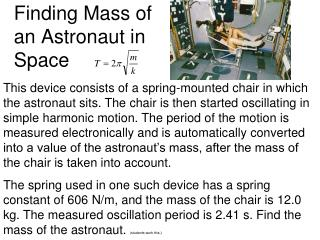DownloadDownload PresentationFinding Mass of an Astronaut in Space

# Finding Mass of an Astronaut in Space

Download Presentation## Finding Mass of an Astronaut in Space

- - - - - - - - - - - - - - - - - - - - - - - - - - - E N D - - - - - - - - - - - - - - - - - - - - - - - - - - -
##### Presentation Transcript

1. Finding Mass of an Astronaut in Space This device consists of a spring-mounted chair in which the astronaut sits. The chair is then started oscillating in simple harmonic motion. The period of the motion is measured electronically and is automatically converted into a value of the astronaut’s mass, after the mass of the chair is taken into account. The spring used in one such device has a spring constant of 606 N/m, and the mass of the chair is 12.0 kg. The measured oscillation period is 2.41 s. Find the mass of the astronaut. (students work this.)

2. Mass of an Astronaut

3. Example 1 The Wavelengths of Radio Waves AM and FM radio waves are transverse waves consisting of electric and magnetic field disturbances traveling at a speed of 3.00x108m/s. (What is this number?) A station broadcasts AM radio waves whose frequency is 1230x103Hz and an FM radio wave whose frequency is 91.9x106Hz. Find the distance between adjacent crests in each wave.

4. 16.2 Periodic Waves Example 1 The Wavelengths of Radio Waves AM and FM radio waves are transverse waves consisting of electric and magnetic field disturbances traveling at a speed of 3.00x108m/s. A station broadcasts AM radio waves whose frequency is 1230x103Hz and an FM radio wave whose frequency is 91.9x106Hz. Find the distance between adjacent crests in each wave.

5. 16.2 Periodic Waves AM FM

6. Problem • Light travels at 3 * 10 8 m/s. Sound travels at 331 m/s. • If lightning strikes 8.2km away from you, how long passes before you see it? • How long passes until you hear it? • (students work this.)

7. Problem • Light travels at 3 * 10 8 m/s. Sound travels at 331 m/s. • V = d/t => t = d/v • If lightning strikes 8.2km away from you, how long passes before you see it? • t = d/v = 8200m / 3 * 10 8 m/s • How long passes until you hear it? • t = d/v = 8200m / 331 m/s • (students work this.)

8. Problem • So you see lightning hit 1 mile away, how many seconds do you count before you hear it? • (students work this.)

9. Problem • So you see lightning hit 1 mile away, how many seconds do you count before you hear it? • t = d/v = 1609m / 331 m/s • So each 5 sec is about 1 mile away. • Each 1 sec is about 3 football fields away. • (Talk about military timing of artillery and nukes.)

10. THE PRINCIPLE OF LINEAR SUPERPOSITION When two or more waves are present simultaneously at the same place, the resultant disturbance is the sum of the disturbances from the individual waves.

11. Constructive Interference • Two waves, a and b, have the same frequency and amplitude • Are in phase • The combined wave, c, has the same frequency and a greater amplitude

12. Destructive Interference • Two waves, a and b, have the same amplitude and frequency • They are half a cycle out of phase • When they combine, the waveforms cancel

13. Reflection of Waves – Fixed End • Whenever a traveling wave reaches a boundary, some or all of the wave is reflected

14. Reflection of Waves – Fixed End • Whenever a traveling wave reaches a boundary, some or all of the wave is reflected • When it is reflected from a fixed end, the wave is inverted • The shape remains the same

15. What happens when a wave interferes with its own reflection? • Sometimes we get standing waves.

16. 1st Harmonic

17. 2nd Harmonic

18. 3rd Harmonic

19. The a string will only allow certain wavelengths to be standing waves. • Because the endpoints need to be nodes. • For a string with two fixed ends, • λ= 2L / n n = 1, 2, 3, 4 …….